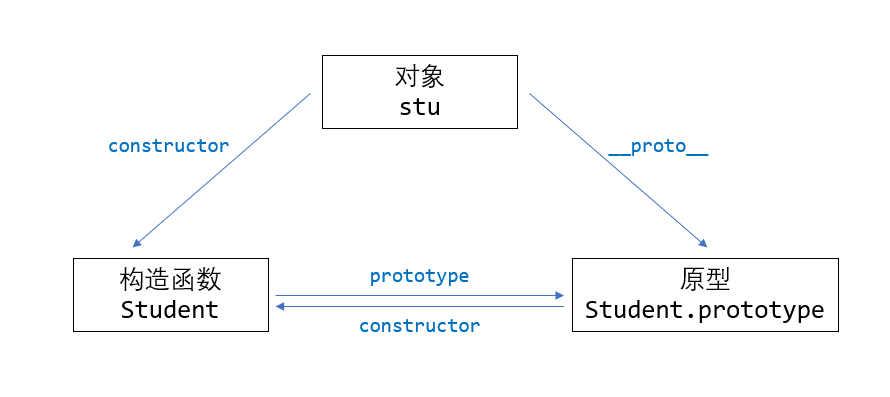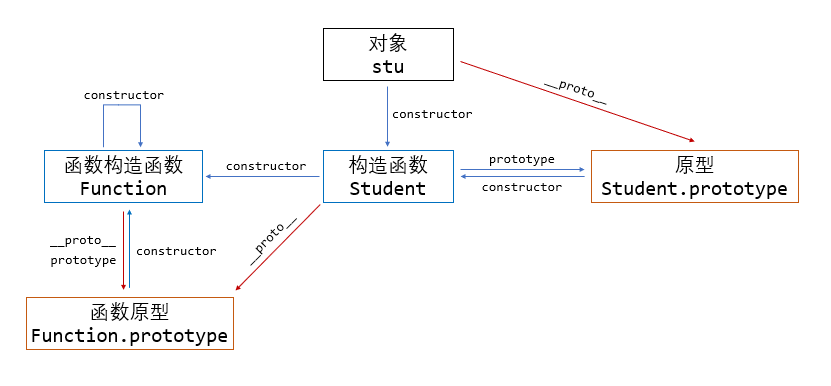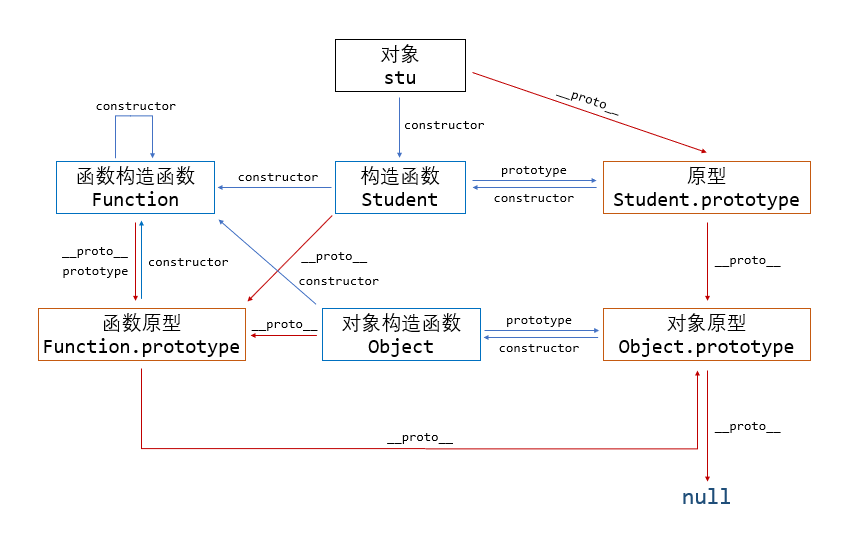# JavaScript 的面向对象进阶

## JavaScript 的面向对象进阶¶

### JavaScript 的第一原则¶

 1 2 3 4 5 6 let a = { foo: 1, bar: "foobar", }; let b = [1, 2, 3]; 

 1 2 3 4 5 6 7 8 let a = new Object(); a.foo = 1; a.bar = "foobar"; let b = new Array(); b = 1; b = 2; b = 3; 

 1 2 typeof Object; // function typeof Array; // function 

### 原型和构造函数¶

 1 2 3 4 5 6 7 8 function createStudent(_name, _age) { let res = {}; res.name = _name; res.age = _age; return res; } let stu = createStudent("Ashitemaru", 22); 

 1 2 3 4 5 6 function Student(_name, _age) { this.name = _name; this.age = _age; } let stu = new Student("Ashitemaru", 22); 

 1 2 3 4 5 6 7 8 9 function Student(_name, _age) { this.name = _name; this.age = _age; } let stu = Student("Ashitemaru", 22); stu; // undefined name; // Ashitemaru 

 1 2 3 4 5 6 function sum(x, y) { return x + y; } let num = sum(1, 2); // 3 let obj = new sum(1, 2); // {} 

 1 2 3 4 5 6 7 8 function Student(_name, _age) { this.name = _name; this.age = _age; this.study = () => { console.log("studying..."); }; } 

 1 2 3 Student.prototype.study = () => { console.log("studying..."); }; 

 1 2 3 const stu = new Object(); stu.__proto__ = Student.prototype; Student.call(stu, "Ashitemaru", 22); 

  1 2 3 4 5 6 7 8 9 10 function Student(_name, _age) { this.name = _name; this.age = _age; } Student.prototype.study = () => { console.log("studying..."); }; let stu = new Student("Ashitemaru", 22); stu.study(); // studying... 

 1 stu.__proto__ === Student.prototype; // true 

__proto__ 属性是所有对象都具有的，其指向这个对象的原型，也就是这个对象构造函数 prototype 所指向的对象。

__proto__ 是即将废弃的属性，并且和 Python 类似，其名称使用双下划线包围实际上就代表了其理应私有，不能直接访问。

 1 stu.constructor === Student; // true 

 1 Student.prototype.constructor === Student; // true1 2 3 4 5 6 7 8 9 10 class Student { constructor(_name, _age) { this.name = _name; this.age = _age; } study() { console.log("studying..."); } } 

  1 2 3 4 5 6 7 8 9 10 11 var Student = /** @class */ (function () { function Student(_name, _age) { this.name = _name; this.age = _age; } Student.prototype.study = function () { console.log("studying..."); } return Student; }()); 

### 函数的原型¶

JavaScript 的函数的包装类为 FunctionFunction 是函数的构造函数，所以实际上声明函数还有一种写法：

 1 2 const sum = new Function("x", "y", "return x + y"); sum(1, 2); // 3 

 1 2 3 function foo() { } foo.__proto__ === Function.prototype; // true 

 1 2 Object.__proto__ === Function.prototype; // true Function.__proto__ === Function.prototype; // true 

 1 2 3 4 function foo() { } foo.constructor === Function; // true Function.constructor === Function; // true### 原型的原型¶

JavaScript 认为所有的原型均是由 Object 直接产生的，也就是说和 new Object() 创建出来的无异，只不过后续增添若干属性。在这样的设计思想下，所有原型的 __proto__ 均指向 Object.prototype。但有个例外，Object.prototype 也是原型，但是其 __proto__ 指向 null。也就是说：Note

### 继承¶

#### 原型链继承¶

  1 2 3 4 5 6 7 8 9 10 11 12 13 14 15 16 17 18 19 20 21 22 23 24 25 26 27 28 29 30 31 32 33 34 35 36 37 38 39 40 // 让我们从一个函数里创建一个对象 o，它自身拥有属性 a 和 b 的： function f() { this.a = 1; this.b = 2; } let o = new f(); // {a: 1, b: 2} // 在 f 函数的原型上定义属性 f.prototype.b = 3; f.prototype.c = 4; // 不要在 f 函数的原型上直接定义 f.prototype = {b:3,c:4};这样会直接打破原型链 // o.[[Prototype]] 有属性 b 和 c // (其实就是 o.__proto__ 或者 o.constructor.prototype) // o.[[Prototype]].[[Prototype]] 是 Object.prototype. // 最后 o.[[Prototype]].[[Prototype]].[[Prototype]] 是 null // 这就是原型链的末尾，即 null， // 根据定义，null 就是没有 [[Prototype]]。 // 综上，整个原型链如下： // {a:1, b:2} ---> {b:3, c:4} ---> Object.prototype---> null console.log(o.a); // 1 // a 是 o 的自身属性吗？是的，该属性的值为 1 console.log(o.b); // 2 // b 是 o 的自身属性吗？是的，该属性的值为 2 // 原型上也有一个'b'属性，但是它不会被访问到。 // 这种情况被称为"属性遮蔽 (property shadowing)" console.log(o.c); // 4 // c 是 o 的自身属性吗？不是，那看看它的原型上有没有 // c 是 o.[[Prototype]] 的属性吗？是的，该属性的值为 4 console.log(o.d); // undefined // d 是 o 的自身属性吗？不是，那看看它的原型上有没有 // d 是 o.[[Prototype]] 的属性吗？不是，那看看它的原型上有没有 // o.[[Prototype]].[[Prototype]] 为 null，停止搜索 // 找不到 d 属性，返回 undefined 

JavaScript 并没有其他基于类的语言所定义的“方法”。在 JavaScript 里，任何函数都可以添加到对象上作为对象的属性。函数的继承与其他的属性继承没有差别，包括上面的“属性遮蔽”（这种情况相当于其他语言的方法重写）。

  1 2 3 4 5 6 7 8 9 10 function Student(_name, _age) { this.name = _name; this.age = _age; } Student.prototype.study = () => { console.log("studying..."); }; let stu = new Student("Ashitemaru", 22); stu.toString(); // '[object Object]' 

  1 2 3 4 5 6 7 8 9 10 11 12 13 14 15 16 17 18 19 20 21 22 let Person = /** @class */ (function () { function Person(_name, _age) { this.name = _name; this.age = _age; } Person.prototype.walk = function () { console.log("walking..."); } return Person; }()); let Student = /** @class */ (function () { function Student(_school) { this.school = _school; } Student.prototype.study = function () { console.log("studying..."); } return Student; }()); 

 1 2 3 4 5 // before stu --> Student.prototype --> Object.prototype --> null // after stu --> Student.prototype(per) --> Person.prototype --> Object.prototype --> null 

  1 2 3 4 5 6 7 8 9 10 11 12 13 14 15 16 17 18 19 20 21 22 23 24 25 26 27 28 29 30 31 32 33 let Person = /** @class */ (function () { function Person(_name, _age) { this.name = _name; this.age = _age; this.val = ; // Only for display } Person.prototype.walk = function () { console.log("walking..."); } return Person; }()); let Student = /** @class */ (function () { function Student(_school) { this.school = _school; } let per = new Person("Ashitemaru", 22); Student.prototype = per; // Reset prototype Student.prototype.study = function () { console.log("studying..."); } return Student; }()); let stu = new Student("Tsinghua"); stu.walk(); // walking... stu.study(); // studying... console.log(stu.name, stu.school, stu.age); // Ashitemaru Tsinghua 22 

 1 2 3 4 5 let stu1 = new Student("Tsinghua"); let stu2 = new Student("Peking"); stu1.val.push(2); console.log(stu2.val); // [1, 2] 

#### 借用构造继承¶

  1 2 3 4 5 6 7 8 9 10 11 12 13 14 15 16 17 18 19 20 21 22 23 24 25 26 27 28 29 30 31 32 33 34 35 let Person = /** @class */ (function () { function Person(_name, _age) { this.name = _name; this.age = _age; this.val = ; // Only for display this.walk = function () { console.log("walking..."); } // Must define class methods inside the constructor // instead of defining them in prototype } Person.prototype.run = function() { console.log("running..."); } return Person; }()); let Student = /** @class */ (function () { function Student(_name, _age, _school) { Person.call(this, _name, _age); this.school = _school; } Student.prototype.study = function () { console.log("studying..."); } return Student; }()); let stu = new Student("Ashitemaru", 22, "Tsinghua"); stu.walk(); // walking... stu.study(); // studying... console.log(stu.name, stu.school, stu.age); // Ashitemaru Tsinghua 22 

 1 2 let stu = new Student("Ashitemaru", 22, "Tsinghua"); stu.run; // undefined 

 1 2 3 4 5 let stu1 = new Student("Ashitemaru", 22, "Tsinghua"); let stu2 = new Student("SmileLee", 22, "Peking"); stu1.val.push(2); console.log(stu2.val); //  

 1 stu1.walk === stu2.walk; // false 

#### 组合继承¶

  1 2 3 4 5 6 7 8 9 10 11 12 13 14 15 16 17 18 19 20 21 22 23 24 25 26 27 28 29 30 31 32 33 34 let Person = /** @class */ (function () { function Person(_name, _age) { this.name = _name; this.age = _age; this.val = ; // Only for display } // Keep unshareable variables in constructor Person.prototype.walk = function () { console.log("walking..."); } // Keep shareable variables in prototype return Person; }()); let Student = /** @class */ (function () { function Student(_name, _age, _school) { Person.call(this, _name, _age); this.school = _school; } let per = new Person(); Student.prototype = per; // Reset prototype Student.prototype.study = function () { console.log("studying..."); } return Student; }()); let stu = new Student("Ashitemaru", 22, "Tsinghua"); stu.walk(); // walking... stu.study(); // studying... console.log(stu.name, stu.school, stu.age); // Ashitemaru Tsinghua 22 

 1 2 3 4 5 6 7 8 let stu1 = new Student("Ashitemaru", 22, "Tsinghua"); let stu2 = new Student("SmileLee", 22, "Peking"); stu1.val.push(2); console.log(stu2.val); //  stu1.walk === stu2.walk; // true stu1.walk === undefined; // false 

#### 寄生组合继承¶

 1 2 3 4 5 // before stu --> Student.prototype --> Object.prototype --> null // after stu --> Student.prototype(per) --> Person.prototype --> Object.prototype --> null 

  1 2 3 4 5 6 7 8 9 10 11 12 13 14 15 16 17 18 19 20 21 22 23 24 25 26 27 28 29 30 31 let Person = /** @class */ (function () { function Person(_name, _age) { this.name = _name; this.age = _age; this.val = ; // Only for display } Person.prototype.walk = function () { console.log("walking..."); } return Person; }()); let Student = /** @class */ (function () { function Student(_name, _age, _school) { Person.call(this, _name, _age); this.school = _school; } function F() { } F.prototype = Person.prototype; Student.prototype = new F(); Student.prototype.constructor = Student; Student.prototype.study = function () { console.log("studying..."); } return Student; }()); 

### 创建对象和生成原型链¶

#### 使用构造器创建的对象¶

  1 2 3 4 5 6 7 8 9 10 11 12 13 14 function Graph() { this.vertices = []; this.edges = []; } Graph.prototype = { addVertex: function(v) { this.vertices.push(v); } }; const g = new Graph(); // g 是生成的对象，他的自身属性有 'vertices' 和 'edges'。 // 在 g 被实例化时，g.[[Prototype]] 指向了 Graph.prototype。 

  1 2 3 4 5 6 7 8 9 10 11 12 function Graph() { this.vertices = []; this.edges = []; } Graph.prototype.addVertex = function(v) { this.vertices.push(v); } const g = new Graph(); // g 是生成的对象，他的自身属性有 'vertices' 和 'edges'。 // 在 g 被实例化时，g.[[Prototype]] 指向了 Graph.prototype。 

  1 2 3 4 5 6 7 8 9 10 11 12 13 14 15 16 17 18 19 20 function GraphInner(type) { this.type = type; } GraphInner.prototype.printType = function () { console.log(this.type); } function Graph() { this.vertices = []; this.edges = []; } Graph.prototype = new GraphInner('Sparse graph'); Graph.prototype.addVertex = function(v){ this.vertices.push(v); } const g = new Graph(); g.printType(); // => Sparse graph 

#### 使用 Object.create 创建对象¶

ECMAScript 5 中引入了一个新方法：Object.create()。可以调用这个方法来创建一个新对象。新对象的原型就是调用 create 方法时传入的第一个参数：

  1 2 3 4 5 6 7 8 9 10 11 12 13 var a = {a: 1}; // a ---> Object.prototype ---> null var b = Object.create(a); // b ---> a ---> Object.prototype ---> null console.log(b.a); // 1 (继承而来) var c = Object.create(b); // c ---> b ---> a ---> Object.prototype ---> null var d = Object.create(null); // d ---> null console.log(d.hasOwnProperty); // undefined，因为 d 没有继承 Object.prototype 

#### 使用 class 关键字创建对象¶

  1 2 3 4 5 6 7 8 9 10 11 12 13 14 15 16 17 18 19 20 21 class Polygon { constructor(height, width) { this.height = height; this.width = width; } } class Square extends Polygon { constructor(sideLength) { super(sideLength, sideLength); } get area() { return this.height * this.width; } set sideLength(newLength) { this.height = newLength; this.width = newLength; } } var square = new Square(2);